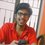# Can we generalize it?

I was working on one question:- if there are 1 red balls, 2 blue balls, 3 green balls, 4 yellow balls and 5 white balls placed on a table, what is the number of ways of selecting 5 out of 5. My process came out with 7 cases as follows:-

• 5 alike balls.
• 4 alike balls and 1 different ball.
• 3 alike balls and 2 different balls.
• 3 alike balls of one colour and 2 alike balls of another colour.
• 2 alike balls and 3 different balls.
• 2 alike balls of one colour, 2 alike balls of another colour and 1 different ball.
• 5 different balls.

I got interested and tried to generalize the number of such cases that would form if we have to select $n$ balls from 1 ball of colour-1, 2 balls of colour-2, 3 balls of colour-3, $\cdots n$ balls of colour $n$.

It turns out that when $n = 1$, the number of cases is $1$.
When $n = 2$, the number of cases is $2$.
When $n = 3$, the number of cases is $3$.
When $n = 4$, the number of cases is $5$.
When $n = 5$, the number of cases is $7$. (as discussed above).
When $n = 6$, the number of cases is $12$.

Now, just in case that the sixth case becomes clear, here are the cases I found:-

• 6 alike.
• 5 alike and 1 different.
• 4 alike and 2 different.
• 4 alike and 2 alike.
• 3 alike and 3 different.
• 3 alike and 2 alike and 1 different.
• 3 alike and 3 alike.
• 2 alike and 4 different.
• 2 alike and 3 alike and 1 different.
• 2 alike and 2 alike and 2 different.
• 2 alike and 2 alike and 2 alike.
• 6 different.

Looking at the pattern, the first thing which came into my mind is the fibonacci sequence, but as you can observe, the pattern is broken at $n = 5$. So, is there any nice method to calculate the number of cases that can be formed?

A detailed solution is appreciated.Note by Ashish Menon
4 years, 10 months ago

This discussion board is a place to discuss our Daily Challenges and the math and science related to those challenges. Explanations are more than just a solution — they should explain the steps and thinking strategies that you used to obtain the solution. Comments should further the discussion of math and science.

When posting on Brilliant:

• Use the emojis to react to an explanation, whether you're congratulating a job well done , or just really confused .
• Ask specific questions about the challenge or the steps in somebody's explanation. Well-posed questions can add a lot to the discussion, but posting "I don't understand!" doesn't help anyone.
• Try to contribute something new to the discussion, whether it is an extension, generalization or other idea related to the challenge.
• Stay on topic — we're all here to learn more about math and science, not to hear about your favorite get-rich-quick scheme or current world events.

MarkdownAppears as
*italics* or _italics_ italics
**bold** or __bold__ bold
- bulleted- list
• bulleted
• list
1. numbered2. list
1. numbered
2. list
Note: you must add a full line of space before and after lists for them to show up correctly
paragraph 1paragraph 2

paragraph 1

paragraph 2

[example link](https://brilliant.org)example link
> This is a quote
This is a quote
    # I indented these lines
# 4 spaces, and now they show
# up as a code block.

print "hello world"
# I indented these lines
# 4 spaces, and now they show
# up as a code block.

print "hello world"
MathAppears as
Remember to wrap math in $$ ... $$ or $ ... $ to ensure proper formatting.
2 \times 3 $2 \times 3$
2^{34} $2^{34}$
a_{i-1} $a_{i-1}$
\frac{2}{3} $\frac{2}{3}$
\sqrt{2} $\sqrt{2}$
\sum_{i=1}^3 $\sum_{i=1}^3$
\sin \theta $\sin \theta$
\boxed{123} $\boxed{123}$

## Comments

Sort by:

Top Newest

Very interesting... Lemme think about it for a bit and see what I can come up with...

- 4 years, 10 months ago

Log in to reply

Sure, take your time. Please notify me if you come up with something. Thanks again! :)

- 4 years, 10 months ago

Log in to reply

@Hung Woei Neoh @Geoff Pilling @Sambhrant Sachan @Sandeep Bhardwaj plz do comment. Special thanks in advance!

- 4 years, 10 months ago

Log in to reply

Your problem is interesting

- 4 years, 10 months ago

Log in to reply

Thanks! Its 1ould great tp know the number of cases that would be rormed before going through making the exhaustive cases, so that we can be sure of our answer. My teacher replied that he needs time to think over it.

- 4 years, 10 months ago

Log in to reply

×

Problem Loading...

Note Loading...

Set Loading...